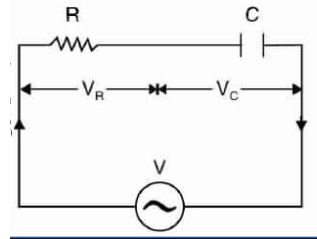# What is RC Series Circuit? Phasor Diagram and Power Curve

0
116

## What is RC Series Circuit?

#### A RL series circuit having resistance R and capacitance C is shown in the figure below.RC SERIES CIRCUIT

#### Let, V = RMS value of applied voltage. I = RMS value of currentVr = Voltage drop across resistance = I*RVc = Voltage drop across capacitance =  I*XcWe know that current in the pure capacitance current leads the voltage by 90 degree. Therefore,current I is 90 degree ahead of Voltage drop across capacitance, Vc.Phasor diagram of series RC circuit is shown in figure.* Voltage drop across R is in phase with the current * Voltage drop across C lags behind current by 90.PHASOR DIAGRAM OF RC SERIES CIRCUIT